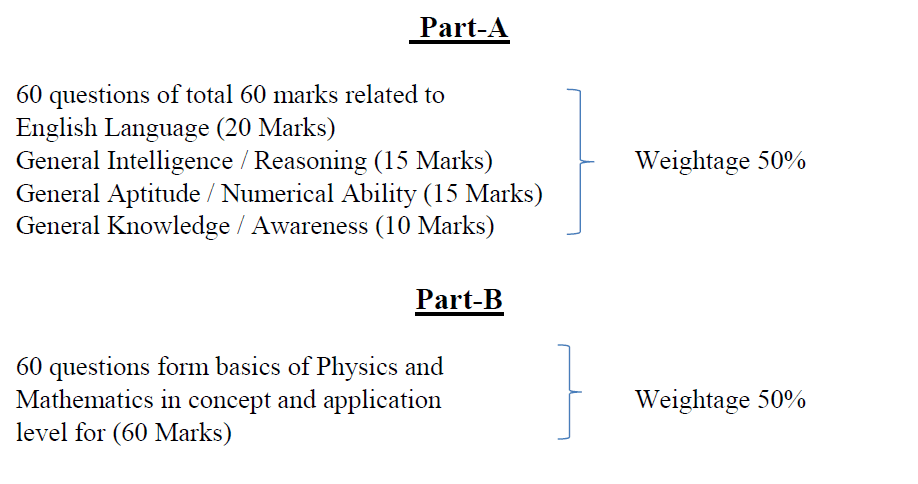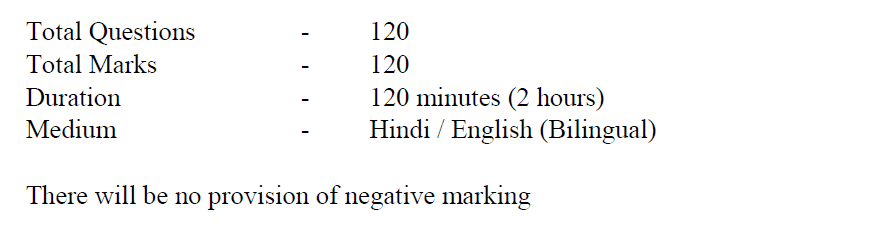# Important/Expected Topics for AAI JE ATC Exam

Hello guys, as we all are aware that AAI is in the process of conducting an online test for the post of Junior Executive -ATC, it is crucial to know what is to be expected in the test. Also as per the syllabus provided by AAI on its official websites, the online test will be comprised of two sections:

• Non-Technical
• Technical## Non-Technical Part:

English Language (20 Questions), General Intelligence/Reasoning (15 questions), General Aptitude/Numerical Ability (15 questions), General Knowledge/ awareness (10 questions). The level of these questions should be moderate, so SSC level books can be referred for the preparation of Non-technical preparation.

## Technical Part:

There will be 30 questions each from Mathematics and Physics. Technical is the main section that decides the fate of almost all candidates appearing in the test as non-tech section is covered well by everyone considering its level. Before writing this post, I went through previous years’ question papers so that whatever I present here is based on that only and not on some kind of speculations

How to Crack ATC Exam in Single Attempt

### The year 2015

Physics:

• Superconductors,
• Scalar and Vectors,
• Satellites time period,
• Newton’s laws of motion (Projectile, Momentum),
• Escape velocity,
• Laws of Thermodynamics,
• Bond structures in solids,
• Carnot cycle,
• wave equations,
• Ampere’s law,
• Gauss law,
• diffraction,
• Occupancy Probability (Fermi-Dirac equation, Bose-Einstein equation),
• Maxwell Equations,
• spring,
• planck’s distribution function,
• London equation,
• Bernoulli Theorem,
• Latent heat,
• Young’s double slit experiment,
• Curie-Weiss Law,
• Capacitance,
• Magnetism,
• Number of modes of vibrations,
• Inductance,
• Torque and Dipole Moment

Mathematics:

• Function,
• Differential Equations,
• Partial Diff. equation,
• Series and its convergence,
• Number of elements,
• Matrices (Rank, system of equations, Eigenvalues),
• Vectors,
• Numerical Methods,
• Residue,
• Sets and subsets,
• Geometry (Line, Ellipse, parabola, planes, Hyperbola, Tangents and normal),
• Indefinite and definite integration,
• Taylor Series.

### The year 2016

Physics:

• Light,
• Lasers,
• X-rays,
• Superconductors,
• Electric and Magnetic Field Equations,
• Curie Temperature,
• Diffraction Experiments and Theories,
• Polarization of waves,
• Interference,
• energy-mass equation,
• pendulum,
• quantum mechanics,
• Theory of relativity,
• Capacitors,
• Work function,
• Uncertainty Principle,
• De Broglie wavelength,
• Newton’s ring experiment,
• Magnetism,
• Poynting Vector

Mathematics:

• Differential Equations (Solution, IF, and CF),
• Set,
• Integration (Definite and indefinite, numerical methods),
• Geometry (Asymptotes, Lines, Tangent, and Normal),
• Limit,
• Matrices (Identity, skew-symmetric, transpose, rank, Inverse),
• Functions (Analytic Function, C.R. equation),
• Convergence of Series,
• Vectors,
• Derivatives (Max., Min. Values),
• Lagrange Theorem and Formula,
• Complex Numbers

### The year 2018

Physics

• Polarization of wave
• Wave Optics
• Ray Optics
• Mechanics
• De Broglie wavelength
• Magnetism
• Properties of diamagnetic, paramagnetic, ferromagnetic substances
• Quantum Mechanics
• Special Theory of Relativity

Mathematics

• Matrices
• Convergence and Divergence of series
• Differential Equation
• Complex Number
• Complex Integral
• Probability
• Definite and Indefinite Integration
• Limits
• Numerical Methods (Bisection, Newton-Raphson methods for finding roots and Trapezoidal Rule of integration, etc.)
• Modern/Abstract Algebra

## Conclusion

So we can conclude that the paper is mainly based on 11-12th Maths and Physics concepts with some topics taken from the Graduation level syllabus. Preparing from IIT preparation notes and NDA previous years’ papers will be very helpful in covering these topics. For graduation level topics, just refer to books of the first year of graduation. I hope covering above mentioned topics will certainly help you get selected in the Airports Authority of India as an Air Traffic Control Officer for a job that is very challenging and stressful but equally rewarding in terms of various allowances paid to ATCOs only.

18 Shares

## Did You Find The Post Useful?

Subscribe Below for More Such Posts

### 18 thoughts on “Important/Expected Topics for AAI JE ATC Exam”

1.Hello sir can I know when can AAI conducts exams FY 2018

1.Most probably in November. I am not sure.

1.Hello sir is there any information about no of posts of ATC Fy2018, As aai hasn’t mentioned in their corriendum updated notification

1.Same as before.

2.Helpful, thank you for the information.

3.Hello Sir,
I read your blog and its really helpful. As this is phase where one should focus on preparation of his/her ATC exam which is to be held on 30th Nov.
But sir there is one question that constantly comes before my eyes while studying,
that What is Expected cutoff this year for ATC this year?
I have also done some exploration regarding this topic and also found some data, but I would like to hear it from your blog.
So I request you to write something on it.
Hope you will write.

1.Cut off depends upon a lot of factors such as difficulty level of question paper which we are yet to see. No doubt no. of students appearing this time are more. There will be more competition. At the same time, since there is no interview, question paper can be designed tougher. So, It’s hard to guess but if you still want a number from us then it’s 80-85. We will definitely post something about cut off after the exam. Best of Luck for your Exam.

4.Is it enough to prepare the NCERT formula based numericals in Physics and Mathematics for the exam?

1.This is the first time when there is no interview. Question paper might be tough. So NCERT alone might not be enough this time.

5.Can you give Ur what’s app number

1.9729649798

1.Sir can you please tell the exam dates of notification 20/21 ATC approximately.

1.March end in normal conditions.

6.Sir, do know the most appropriate online source for preparing JE ATC Exam 2018?

1.Deepankar Sharma

7.Some people are saying that cut off may shoot up to 95…is it is possible????

1.Cut off may even shoot up to 100 if we get easy question paper in exam because no. of posts are less and competition is huge since applications received are much more than any other year. But do we really know the level of difficulty of the exam yet? No. So no matter who is saying what, it’s impossible to predict anything before exam. Considering exam tougher than last year, we are expecting cut off to be around 85.

8.Dear sir please tell me that
Is there interview in 20/21 ATC